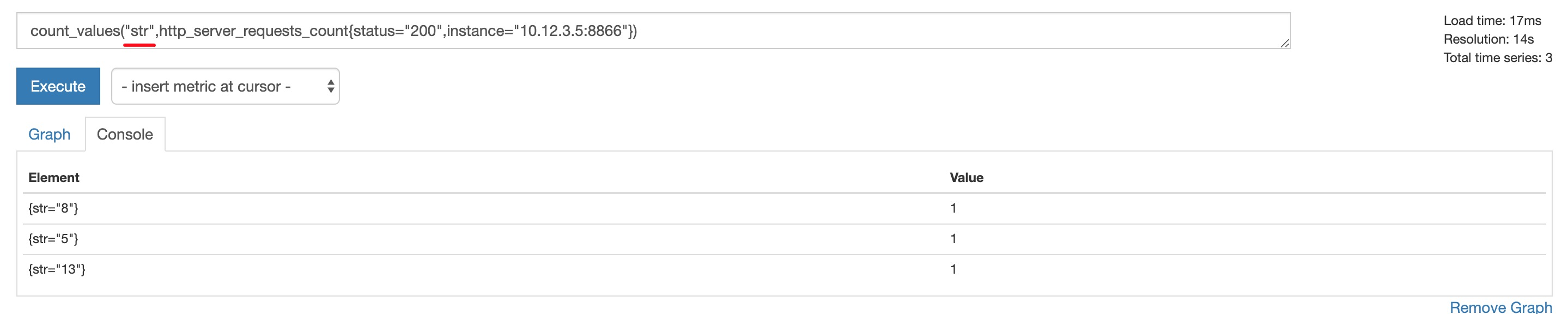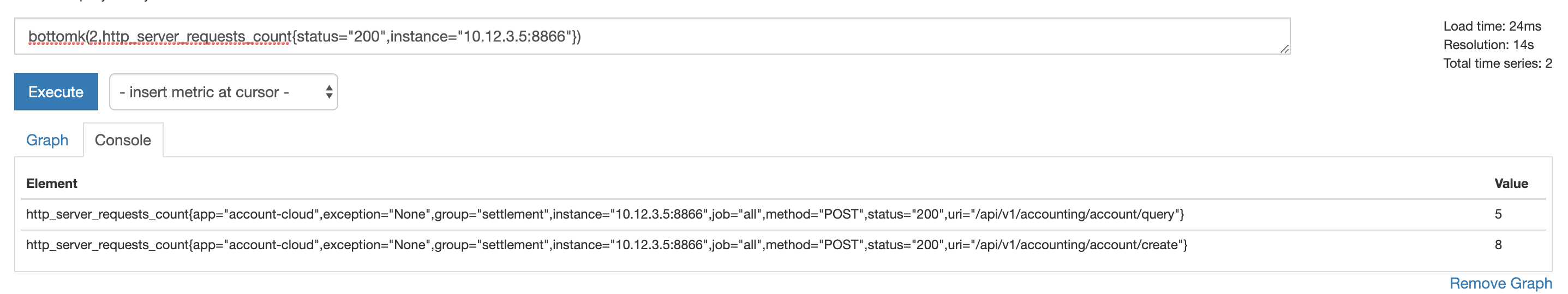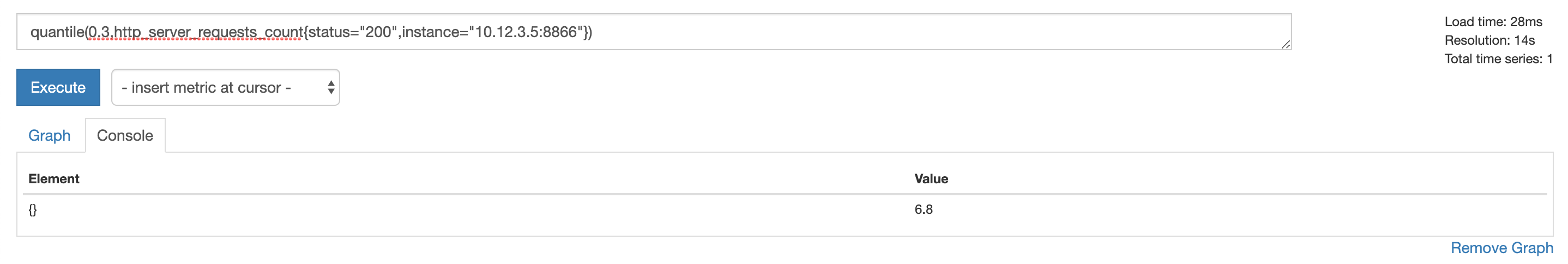# Prometheus 指标查询结果运算

Prometheus 的查询语句支持运算，可以使用二元运算符（算术运算符、比较运算符、集合运算符）和聚合运算符直接操作查询出来的数据集，也可以使用 Prometheus 提供的查询函数进行更复杂的运算。

## vector 的配对问题

1:1，左边 vector 中数据 与 右边 vector 中拥有相同 label 的数据配对，可以用 ignoring 忽略部分 label，或者用 on 指定配对使用的 label，用法如下：

``````<vector expr> <bin-op> ignoring(<label list>) <vector expr>
<vector expr> <bin-op> on(<label list>) <vector expr>
``````

1:N，N:1，group_left 表示右边 vector 中的一个成员会匹配左边的 vector 中的多个成员，group_right 反过来，左边 vector 中的一个成员会匹配右边的 vector 中的多个成员，group 指定的是 N。ignoring 和 on 分别用于删减用于匹配的 label 、指定用于匹配的 label：

``````<vector expr> <bin-op> ignoring(<label list>) group_left(<label list>) <vector expr>
<vector expr> <bin-op> on(<label list>) group_left(<label list>) <vector expr>

<vector expr> <bin-op> ignoring(<label list>) group_right(<label list>) <vector expr>
<vector expr> <bin-op> on(<label list>) group_right(<label list>) <vector expr>
``````

## vector 配对示例

``````method_code:http_errors:rate5m{method="get", code="500"}  24
method_code:http_errors:rate5m{method="get", code="404"}  30
method_code:http_errors:rate5m{method="put", code="501"}  3
method_code:http_errors:rate5m{method="post", code="500"} 6
method_code:http_errors:rate5m{method="post", code="404"} 21

method:http_requests:rate5m{method="get"}  600
method:http_requests:rate5m{method="del"}  34
method:http_requests:rate5m{method="post"} 120
``````

1:1 配对，计算每个方法中 500 错误的占比，因为 method:http_requests:rate5m 没有名为 code 的 label，所以用 ignoring 忽略 code，只剩下 method label 用于配对 ：

``````method_code:http_errors:rate5m{code="500"} / ignoring(code) method:http_requests:rate5m
``````

``````{method="get"}  0.04            //  24 / 600
{method="post"} 0.05            //   6 / 120
``````

N:1 配对，计算每个方法中所有错误码的占比，多个错误代码对应一类请求：

``````method_code:http_errors:rate5m / ignoring(code) group_left method:http_requests:rate5m
``````

``````{method="get", code="500"}  0.04            //  24 / 600
{method="get", code="404"}  0.05            //  30 / 600
{method="post", code="500"} 0.05            //   6 / 120
{method="post", code="404"} 0.175           //  21 / 120
``````

## 二元运算

### 算术运算符

``````+、-、*、/（除法）、%（取模）、^（指数）。
``````

### 比较运算符

``````==、!=、>、<、>=、<=
``````

### 集合运算符

``````and（交集）、or（并集）、unless（差集）
``````

``````http_server_requests_count{status="200"} and http_server_requests_count{method="POST",instance="10.12.3.5:8866"}
``````

``````http_server_requests_count{status="200",method="POST",instance="10.12.3.5:8866"}
``````

vector1 or vector2 是取出 vector1 的所有成员 和 vector2 中不满足 vector1 的筛选条件的成员。

``````## 结果中包含所有满足 method="POST" 的数据，如果重复选择 or 之前的数据。
http_server_requests_count{status="200",instance="10.12.3.5:8866"} or http_server_requests_count{method="POST"}
``````

vector1 unless vector2 取出不满足 vector2 筛选条件的所有 vector1 的成员：

``````http_server_requests_count{status="200",instance="10.12.3.5:8866"} unless http_server_requests_count{method="POST"}
``````

``````http_server_requests_count{status="200",instance="10.12.3.5:8866",method!="POST"}
``````

## 聚合运算

``````<aggr-op>([parameter,] <vector expression>) [without|by (<label list>)]
``````

``````sum:    求和
min:    最小值
max:    最大值
avg:    平均值
stddev: 平方差（stdvar的平方根）
stdvar: 方差
count:  计数
count_values: 统计每个值出现的次数
bottomk: 取结果中最小的 k 位数
topk:    取结果中最大的 k 位数
quantile: 取分位数 (0 ≤ φ ≤ 1）
``````

### 统计每个值出现的次数

``````count_values("str",http_server_requests_count{status="200",instance="10.12.3.5:8866"})
``````### 取前 k 位/后 k 位

``````bottomk(2,http_server_requests_count{status="200",instance="10.12.3.5:8866"})
topk(2,http_server_requests_count{status="200",instance="10.12.3.5:8866"})
``````### 取分位数

``````quantile(0.3,http_server_requests_count{status="200",instance="10.12.3.5:8866"})
``````